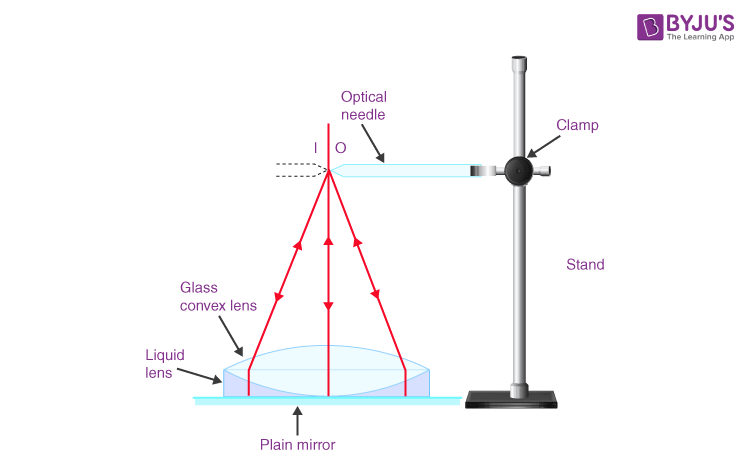# To Find The Refractive Index Of A Liquid By Using A Convex Lens And Plane Mirror

## Aim

To find the refractive index of a liquid using a convex lens and plane mirror.

## Apparatus

• Convex Lens
• Plane Mirror
• Optical Needle
• The clean transparent liquid in a clean beaker
• An iron stand with base and clamp arrangement
• Plane glass slab
• Plumb Line
• Spherometer
• Half metre scale

## Theory

Let us consider f1 and f2 to be the focal length of the glass convex lens and liquid lens respectively and let F be the focal length of their combination, then

$$\begin{array}{l}\frac{1}{F}=\frac{1}{f_{1}}+\frac{1}{f_{2}}\end{array}$$

From the lens maker’s formula

We have,

$$\begin{array}{l}\frac{1}{f_{2}}=(n-1)[\frac{1}{R_{1}}-\frac{1}{R_{2}}]\end{array}$$

Simplifying further, we get

$$\begin{array}{l}\frac{1}{f_{2}}=\frac{(n-1)}{R}\end{array}$$

Inserting values of f2, n can be calculated.

## Diagram## Procedure

### For the focal length of the convex lens,

• Choose a convex lens and find its rough focal length.
• Place the plane mirror on the horizontal base of the iron stand
• Place the convex lens on the plane mirror
• Screw tight the optical needle in the clamp of the stand and hold it horizontally above the lens at a distance equal to its rough focal length.
• Bring the tip of the needle to the principal vertical axis of the lens so the tip of the needle appears to be touching the tip of its image.
• Move the needle up and down and remove the parallax between the tips of the needle and its image.
• Measure the distance between the tip and upper surface of the lens using a plumb line and half metre scale.
• Also, measure the distance between the tip and the surface of its plane mirror.

### For the Focal Length of the Combination

• Take a few drops of transparent liquid on the plane mirror and put a convex mirror over it.
• Repeat 6, 7 and 8.

### (c) For the radius of curvature of the convex lens surface

Observations

1. The rough focal length of convex lens = _____ cm
 Arrangement The distance of Needle Tip Focal Length x (cm) From lens surface x1 (cm) From plane mirror x2 (cm) $$\begin{array}{l}Mean\,x=\frac{x_1+x_2}{2}\end{array}$$ (cm) 1 (2a) (2b) (2c) (3) Without Liquid f1 = _____ With Liquid F = _____

The radius of curvature of the convex lens surface, R = _____ cm.

## Calculations

$$\begin{array}{l}\frac{1}{f_{2}}=\frac{1}{F}-\frac{1}{f_{1}}\end{array}$$

$$\begin{array}{l}n=1+\frac{R}{f_{2}}\end{array}$$

## Precautions

1. Only a few drops of liquid should be taken so as not to thicken the layer.
2. The parallax should be removed tip to tip.
3. The liquid taken should be transparent.

## Sources of Error

1. The liquid may not be quite transparent.
2. The parallax may not be fully removed.

## Viva Voce

1. What is the Refractive Index of a medium?

The ratio of the velocity of light in a vacuum to the velocity of light in the given medium.

2. What are the factors affecting the refractive index of a medium?

The factors affecting the refractive index are

• the nature of the material
• and the wavelength of light used

3. Can the refractive index of a medium be less than or equal to 1?

The Refractive index of a transparent medium is always greater than 1. because the speed of light in any medium is always less than that in a vacuum.

4. What are the laws of reflection?

The laws of reflection state that :

(a) The angle of incidence is always equal to the angle of reflection.

(b) The angle of incidence, angle of refraction and the normal to the point of incidence all lie in one plane.

5. Which substance has the greatest refractive index?

Diamond (2.42) has the greatest refractive index.

Stay tuned to BYJU’S and Fall in Love with Learning!